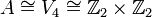# Second cohomology group for trivial group action of Z4 on V4

## Description of the group

We consider here the second cohomology group for trivial group action of cyclic group:Z4 on the Klein four-group, i.e.,$\! H^2(G,A)$

where$G \cong \mathbb{Z}_4$ and$A \cong V_4 \cong \mathbb{Z}_2 \times \mathbb{Z}_2$.

The cohomology group is isomorphic to the Klein four-group.

Note that since cyclic over central implies abelian, all the corresponding group extensions are abelian. Equivalently, all the 2-cocycles are symmetric 2-cocycles.

## Elements

We list here the elements, grouped by similarity under the action of the automorphism groups on both sides.

Cohomology class type Number of cohomology classes Representative 2-cocycle Corresponding group extension Second part of GAP ID (order is 16)
trivial 1$0$ everywhere direct product of Z4 and V4 10
nontrivial 3 direct product of Z8 and Z2 5